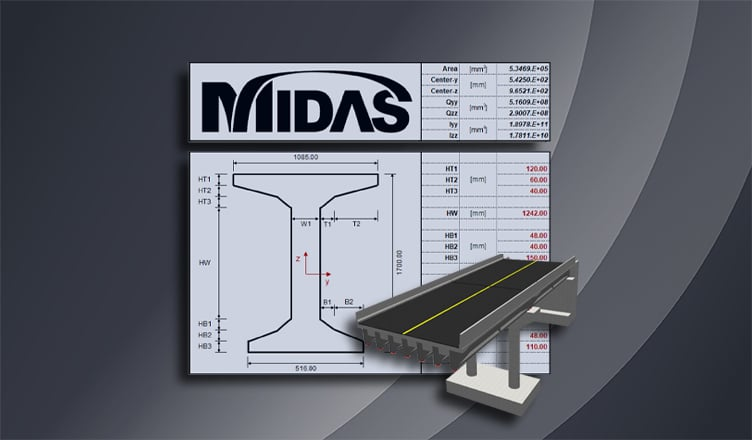BLOG BRIDGE INSIGHT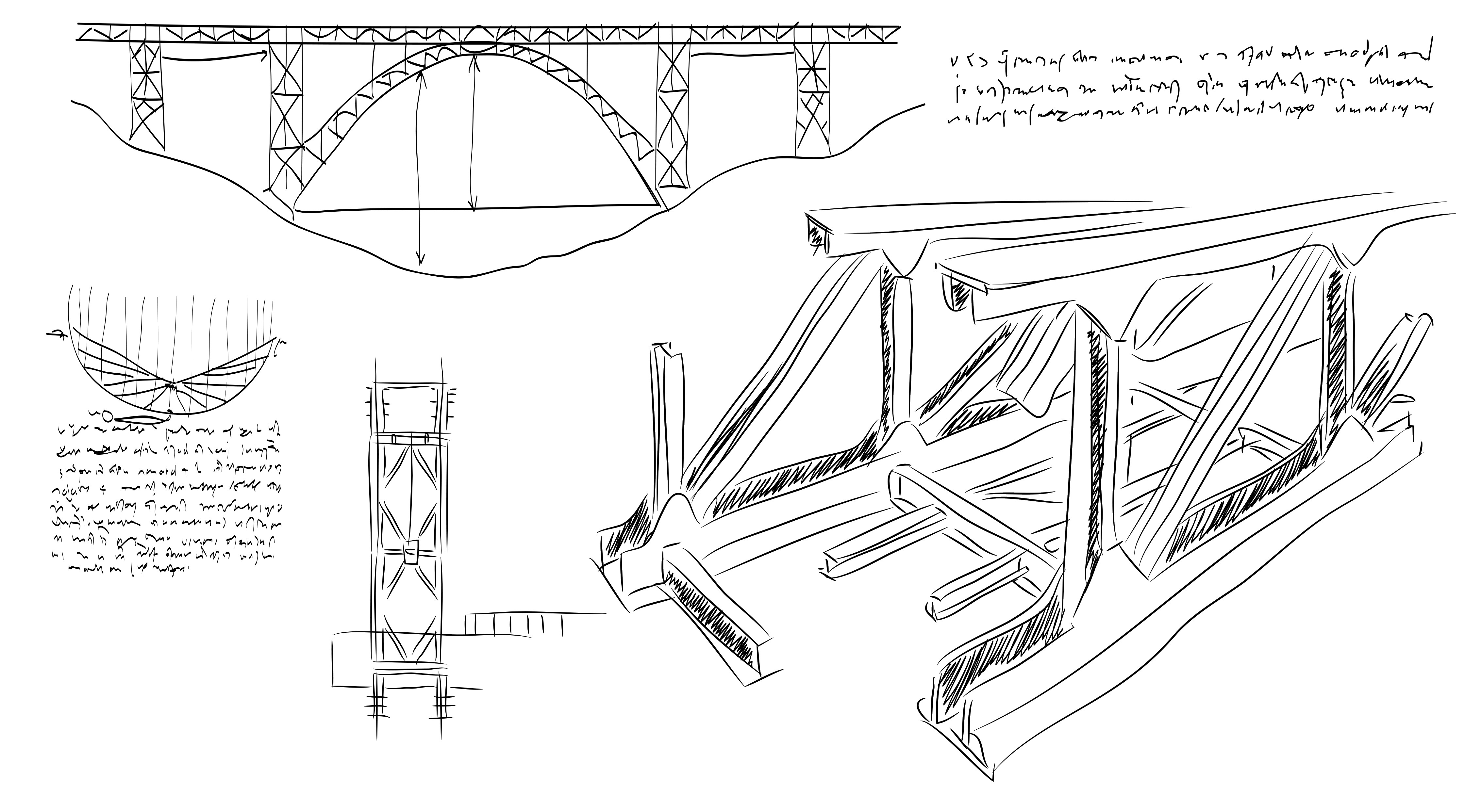The geometric properties of sections, which are indicators of the structural performance and load resistance capacity of sections, are characterized by the section shape and dimensions, regardless of material properties. These section properties are needed to calculate the bending/shear stresses, deflections, strength, and buckling, which are essential factors for the overall structural design of bridges.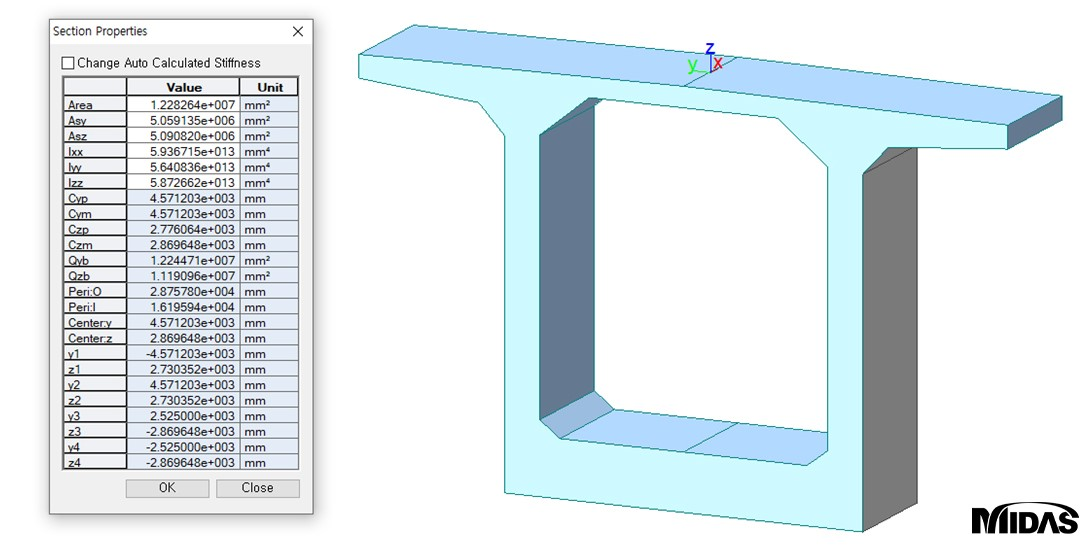Figure 1: Properties of Box Section

Among the section properties, let us look at the relationship between the of an area, the first moment of area, the moment of inertia used in flexure and shear formulas, and how to calculate them.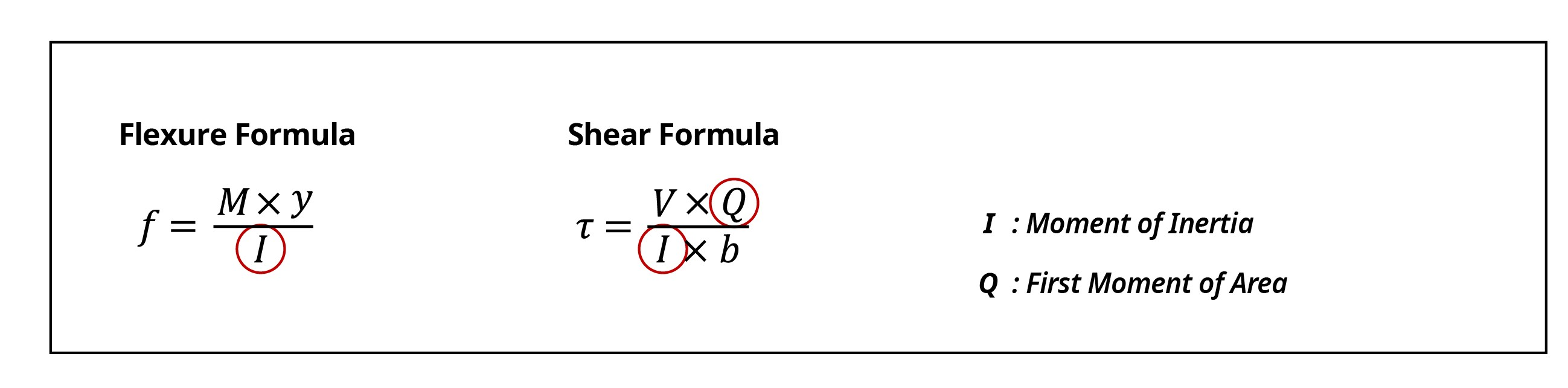Centroid of an Area & First Moment of Area

The first moment of area is mainly used to calculate the centroid of sections and the shear stress of beams, and the formula can be derived through the following process.

The linear region that satisfies Hooke’s Law is confirmed through the stress-strain diagram. The stress-strain relationship of a material with a linear elastic behavior can be expressed as follows along with the strain-curvature relation.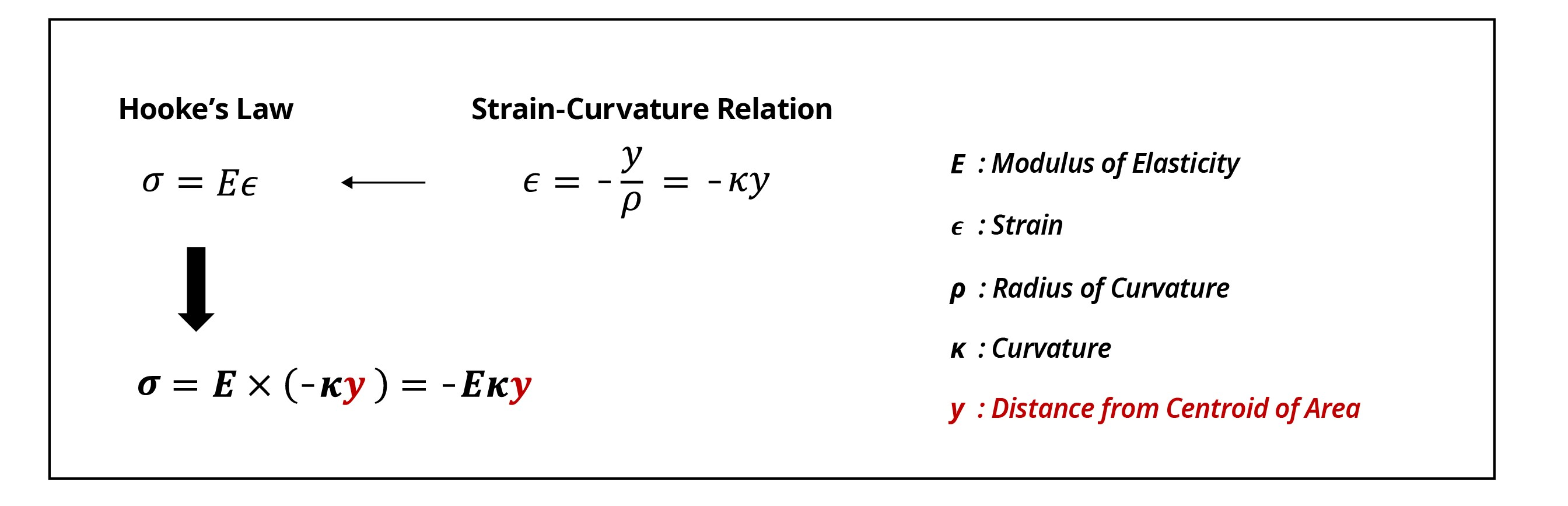By applying the above expression to a section where only flexure acts without axial forces, as shown in Figure 2, one can obtain an expression that calculates the first moment of area. Therefore, the sum of the normal stresses is zero because only flexure acts without axial forces.

The stress ‘σ1’ acting on an element with a distance ‘y’ from the neutral axis and infinitesimal area ‘dA’ within the section can be calculated through the expression above. The sum of the stresses acting on n-infinitesimal areas distributed throughout the entire section is 0, and can be expressed as follows. Since the curvature κ and the elastic modulus E are non-zero constants, the integral of the product of dA and y must be 0.

From this calculation, it can be seen that in the case of linear elastic materials, the neutral axis becomes the centroid of the section and the first moment of area with respect to the centroid is 0.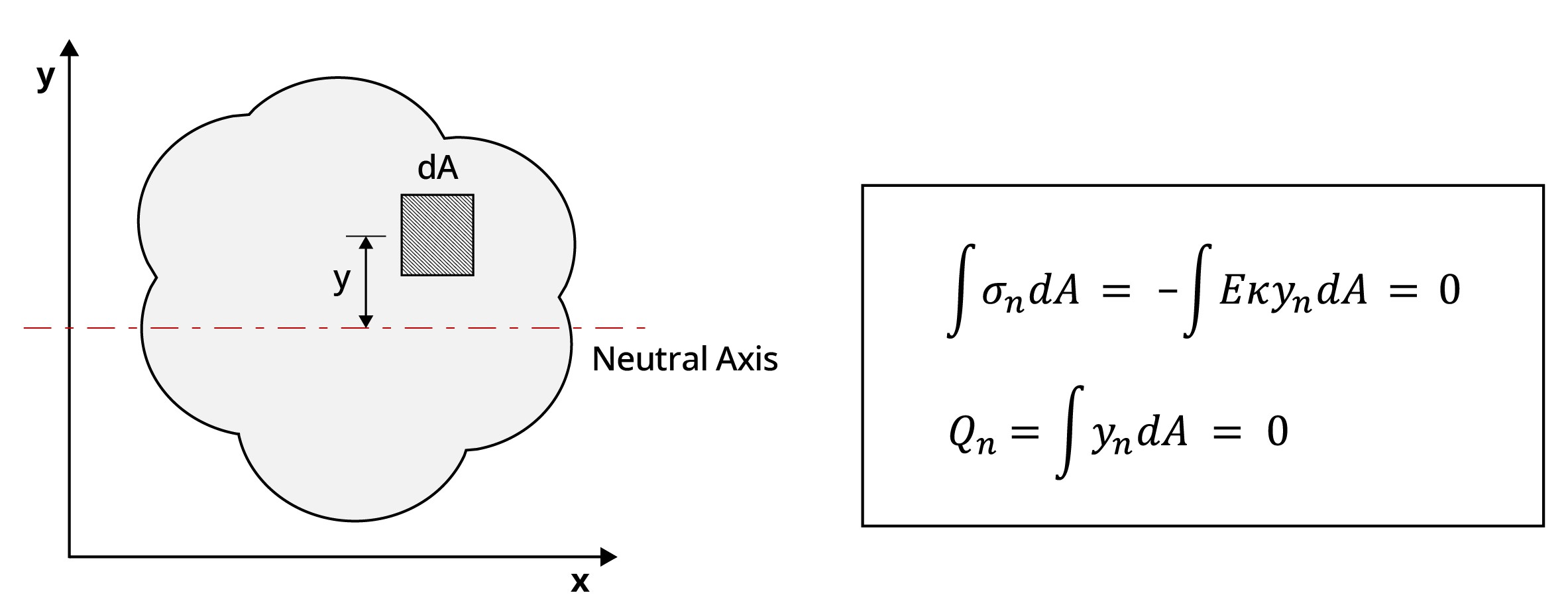Figure 2: Derivation of the First Moment of Area Formula

Using the expression obtained above, the first moment of area and centroid location for the following cases, with the x-axis as their reference axis, can be calculated.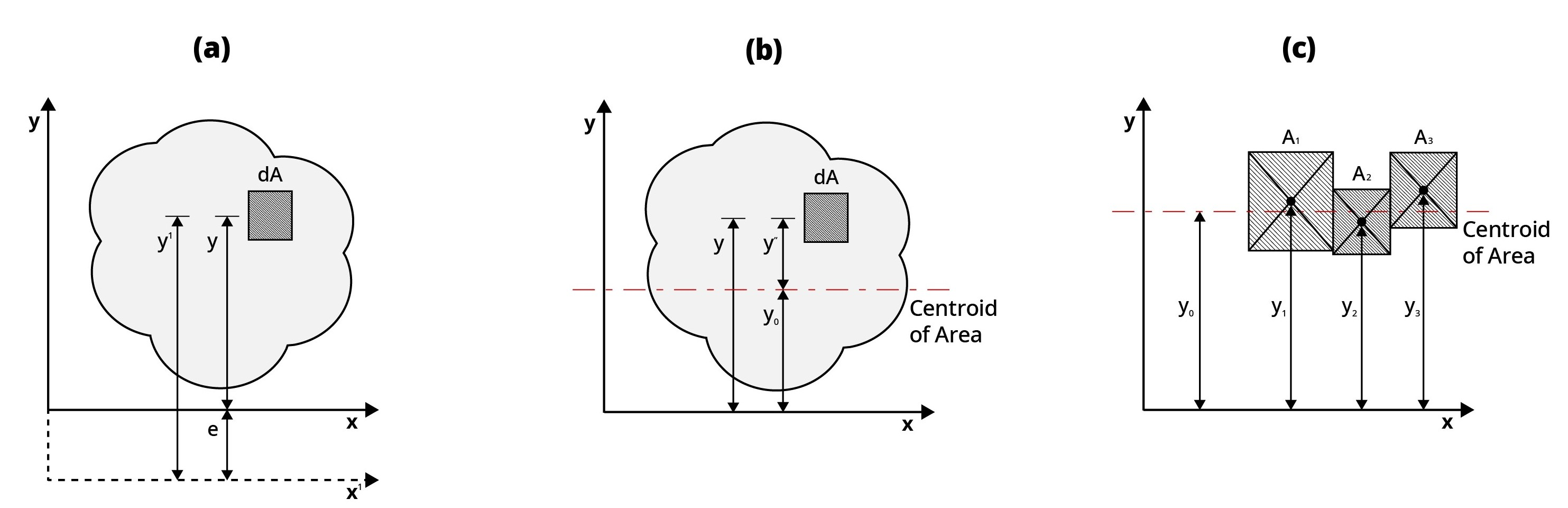Figure 3: Calculating the First Moment of Area for Any Section

• Case (a)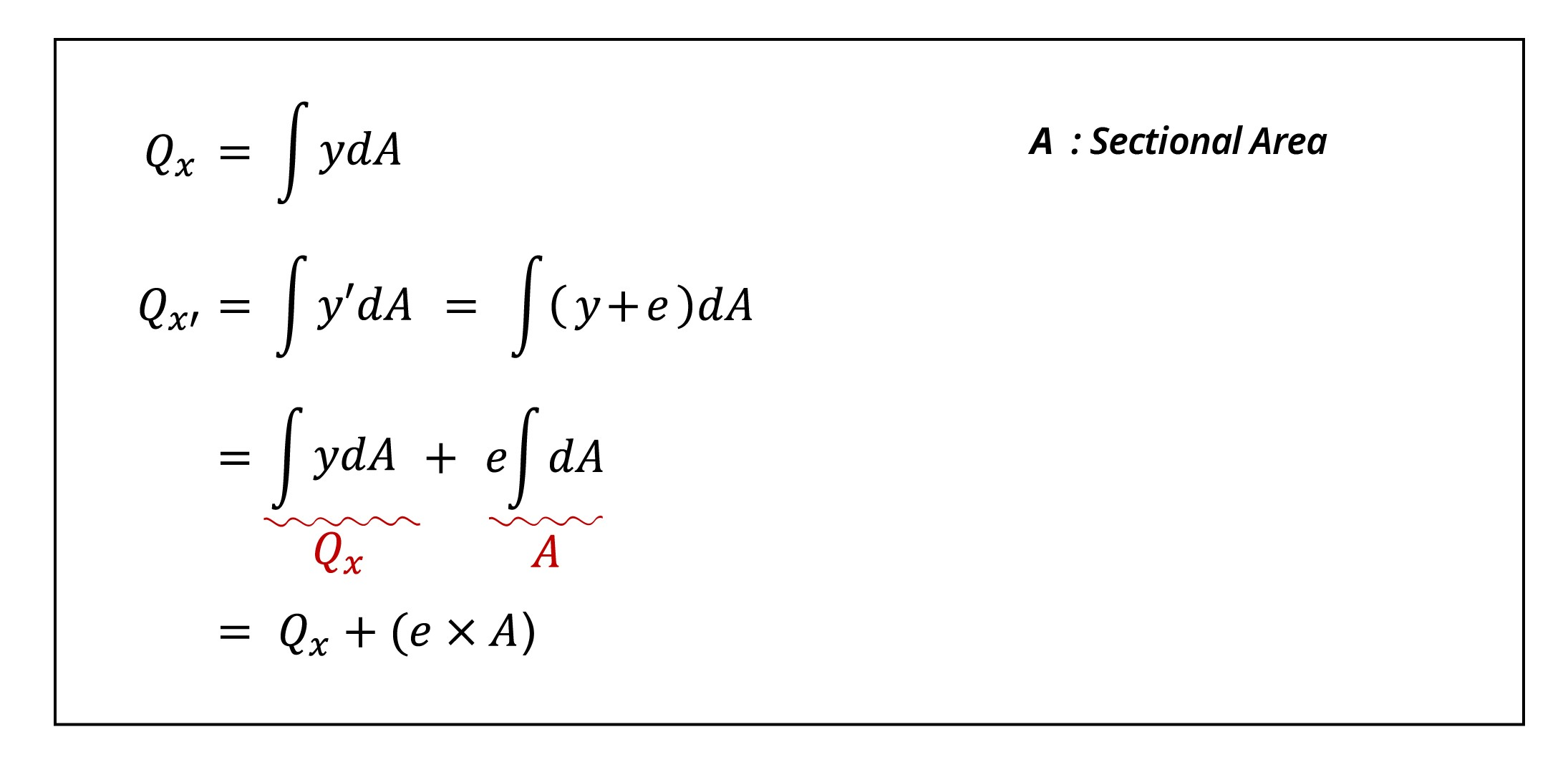• Calculate the first moment of area based on the x’-axis located a distance e from the reference axis x. From the results, it can be seen that, as the distance from the reference axis increases, the first moment of area increases by e.
• The distance y’, which is the distance from the x’-axis to the corresponding element, can be considered separately as y and e.
• Case (b)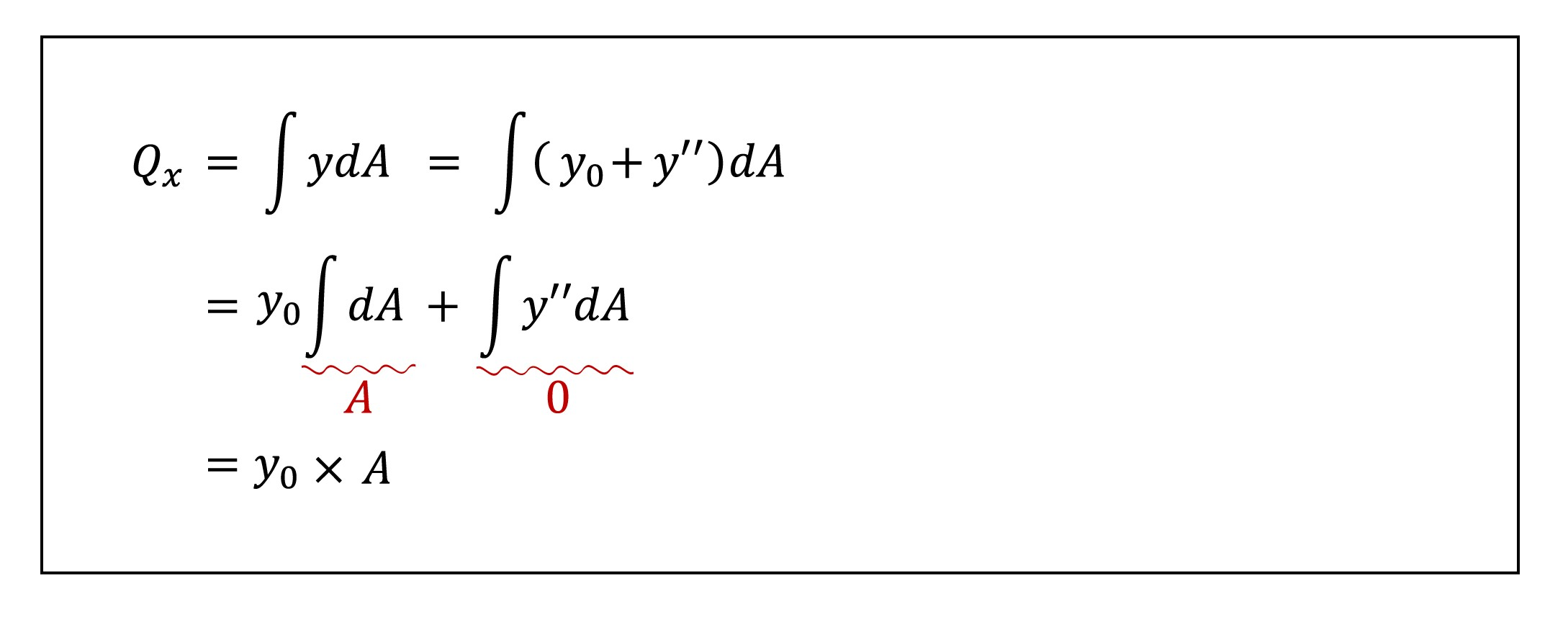• For case (b), the x-axis is set as the reference axis, and the first moment of area can be calculated by separating y into y0, which is the distance from x to the centroid, and y’’, which is the distance from the centroid to the arbitrary area.
• Since the first moment of area from the centroid is 0, y” becomes 0.
• Therefore, the first moment of area is calculated by taking the total cross-sectional area times the distance from the x-axis to the centroid.
• It can be inferred that the first moment of area can be calculated by multiplying the total cross-sectional area by the distance from the reference axis to the centroid.

• Case (c)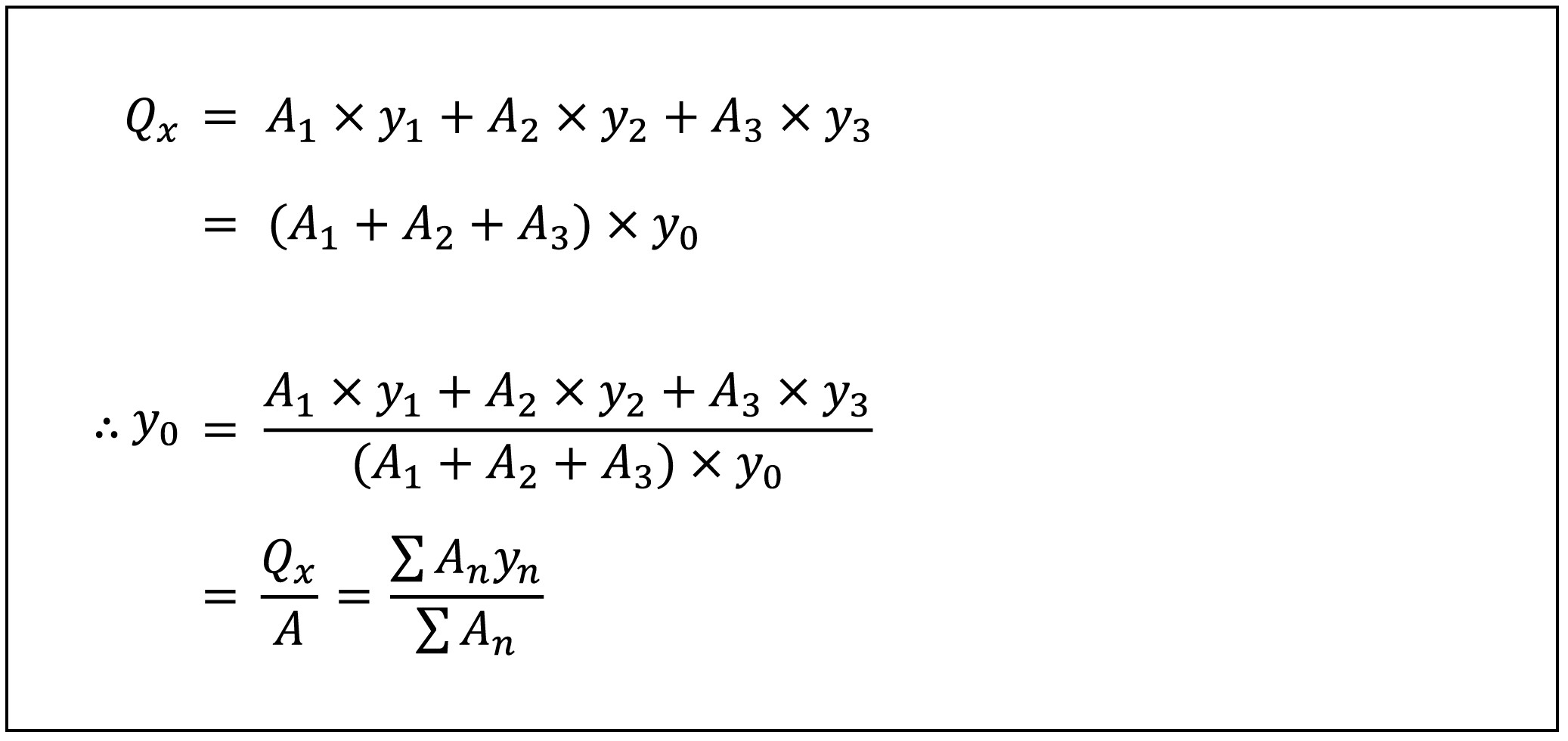• The first moment of area can be calculated by adding the product of the area of each section and the distance from the x-axis to the centroid of the section.
• When knowing the centroid location when the three sections are combined, the first moment of area can be calculated as the product of the total cross-sectional area and y0 as seen in case(b).
• From this result, it is possible to generalize and expression to find the centroid through the first moment of area.

The generalized centroid formula obtained from the above processes can be compared with the results of directly calculating the centroid through the equilibrium conditions. The method of calculating the distance y0 from the reference axis to the centroid G is as follows: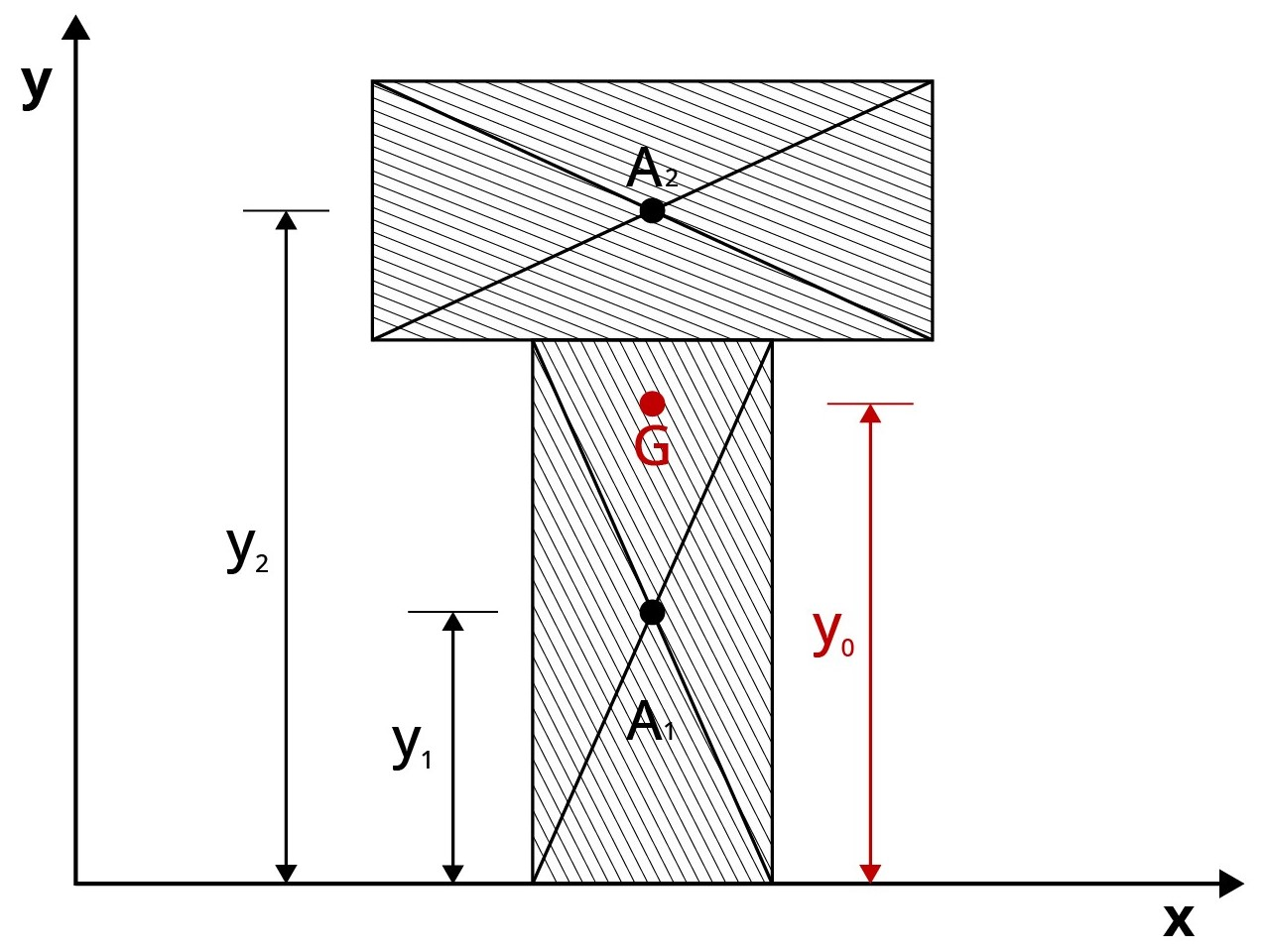Figure 4: Centroid formula using equilibrium equations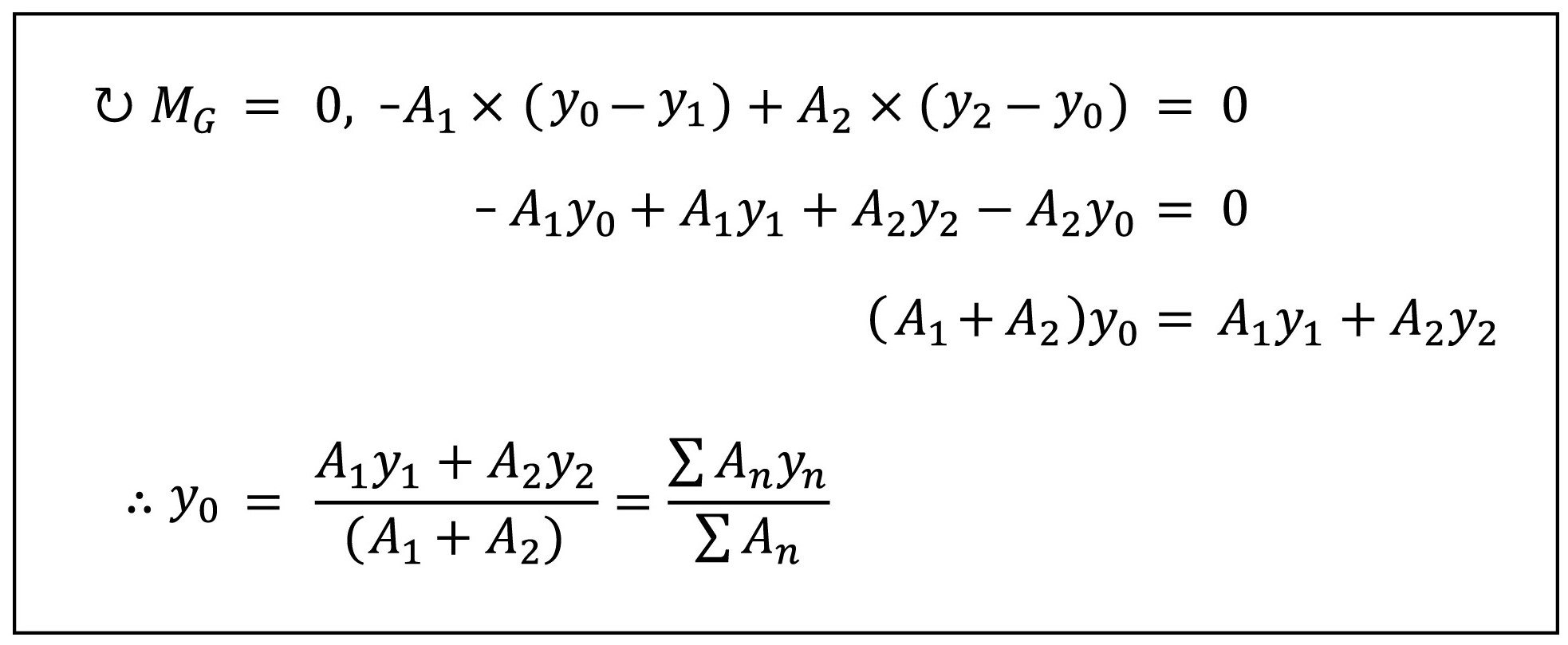The centroid formula obtained through the equilibrium conditions is the same as the formula obtained through the first moment of area.

Example: I-Section Properties

Please look under the DOWNLOAD section below to download "midasBridge I-Section Properties Calculator" excel template. We have provided an easy-to-use calculator where you can enter the desired I-section dimensions and get the results for the centroid, first moment of area, and moment of inertia of I-sections. Moment of inertia will be looked upon in Geometric Properties of Sections: Part II.Kim Min Tae | Technical Engineer | MIDAS IT HQ

Min Tae is a Technical Bridge Engineer within MIDAS IT Technical Planning Team in South Korea. He has over 7+ years of experience in the design of bridges and civil structures. He is also familiar with international codes of practice including AASHTO, Eurocode and BS code.